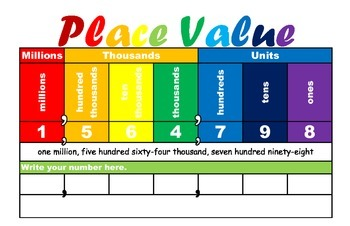•

Unit1: Numbers and Operations in Base TenConcept 1: Place Value
Lesson Essential Questions:
1. How are place values related?

-Base Ten
-Place Value
-Changing a digit within a place value

2. How do I read and write whole numbers in different ways?

- Number forms

3. How do I use symbols to compare numbers?
- Compare and order numbers
- Between forms and expressions

Concept 2: Rounding and Estimation

Lesson Essential Questions:

4. How do I round whole numbers to a given place value?

- Round to Estimate

-Between Forms

- Multi-Step Rounding :(Start with Rounded Number, Work Backwards Round 10 then 100 then 1,000)

5. When is it appropriate to estimate versus calculate?

-ReasonablenessConcept 3: Add and Subtract Multi-digit Numbers

Lesson Essential Question:

6. How do I add and subtract multi-digit numbers?

(Estimating Sums, Commutative, Associative, and Distributive Properties, Partial Sums, Traditional Regrouping)

-Subtract Whole Numbers

(Estimating Differences, Partial Differences, Traditional Regrouping)

- Problem Solving

Concept: Multiplication

Lesson Essential Questions:

7. How do I multiply numbers?

-Estimating Products

- Model Multiplication

- Place Value/Fact Extensions

- Properties

- Partial Products

- Two-Digit Numbers

Concept: Division

Lesson Essential Questions:

8. How do I divide numbers?

-Estimating Quotients

- Model Division

- Place Value/Fact Extensions

- Properties

- Partial Quotients